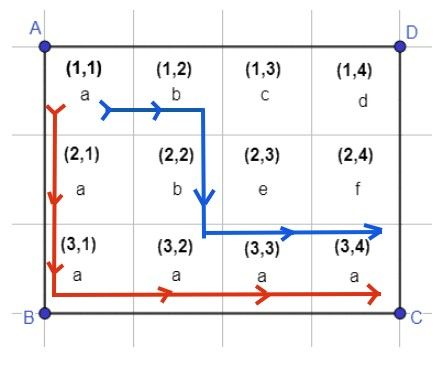# Practice on Toph

Participate in exhilarating programming contests, solve unique algorithm and data structure challenges and be a part of an awesome community.

# No More Child Labor

By Hasinur_ · Limits 2s, 512 MB

Child labor is a global crisis. As you are a problem solver, you need to be aware that many children like you are deprived of education and are being forced to go to work. This is a real-life problem you have to solve in the near future when you will be a great programmer and impactful to the society around you.

Here I will tell you the story of Dipta. He works at a factory far away from his home. He needs to feed his physically disabled mother and his little sister. Dipta lives in a city which can be represented by a grid of $N\times M$ size. Each cell contains a lowercase English alphabet. He lives in the $\mathbf{(1, 1)}$ cell and goes to work at $\mathbf{(N, M)}$ cell. He can move from $(x, y)$ cell to $(x+1, y)$ or $(x, y+1)$ cell only. As you can guess, there are many paths from $(1, 1)$ to $(N, M)$ cell.

Dipta likes to take good paths in his way from home to the workplace.

A path is called a good path if and only if you can make a palindrome string by taking and rearranging the characters found in the path. A palindrome is a word, number, phrase, or other sequence of characters which reads the same backward as forward, such as madam, racecar.

People like you want to establish schools for children like Dipta. Now they want to know how many good paths will contain a school if you establish a school in $(a, b)$ cell.

For better understanding look at the picture below. This is a map of the city of size $N\times M$ where $N = 3$ and $M = 4$.Dipta lives in $(1, 1)$ cell and works at $(3, 4)$ cell.

For example, consider the path: $(1,1) → (1,2) → (2,2) → (3,2) → (3,3) → (3,4)$. Using the letters in the way, Dipta can form the string $\mathbf{''abbaaa''}$ , which can be rearranged to form the palindrome $\mathbf{''aabbaa''}$. So, it is a good path.

If you establish a school at the $(3, 3)$ cell, there will be two good paths that contains the school in the way and they are shown in the picture with red and blue marker.

Now that you are a volunteer to this project, you have to write a computer program to make the task easier. Your program will be given the size of the grid and the letters contained in each cell. For each cell, you have to count the number of good path(s) that will contain that cell. As the answer can be huge, you have to print it modulo $\mathbf{1000000007}$.

## Input

First line of the input file will contain and integer $T$. $T$ denotes the number of testcases.
Each of the test cases start with two integers $N$ and $M$.
Each of the next $N$ lines will contain a string of length $M$.
$j^{th}$ character of the $i^{th}$ line is the character associated with the $(i,j)$ cell.

$\mathbf{Constraints}$

$1 \leq T \leq 100$
$N = 1$
$2 \leq M \leq 10000$

$1 \leq T \leq 100$
$N = 2$
$2 \leq M \leq 10000$

$1 \leq T \leq 10$
$3 \leq N \leq 40$
$3 \leq M \leq 40$

It is guaranteed that all the letters in the grid cells will be within first 10 lowercase letters of the English alphabet.

## Output

For each test case print "Case x:" in the first line without quotation marks, where x is the test case number you are processing now. Then print $N$ lines. Each of those $N$ line will contain $M$ integers separated by space. $j^{th}$ integer of the $i^{th}$ line is the answer for the $(i,j)$ cell. As the required answer can be huge you have to print the answer modulo $\mathbf{1000000007}$.

## Samples

InputOutput
1
1 4
aaaa

Case 1:
1 1 1 1

InputOutput
1
2 5
aaaaa
aaaaa

Case 1:
5 4 3 2 1
1 2 3 4 5

InputOutput
1
3 4
aaaa
aaaa
aaaa

Case 1:
10 6 3 1
4 6 6 4
1 3 6 10


### Statistics

37% Solution Ratio

mohanr7073Earliest, Jul '20

Talha_TakiFastest, 0.1s

Talha_TakiLightest, 14 MB